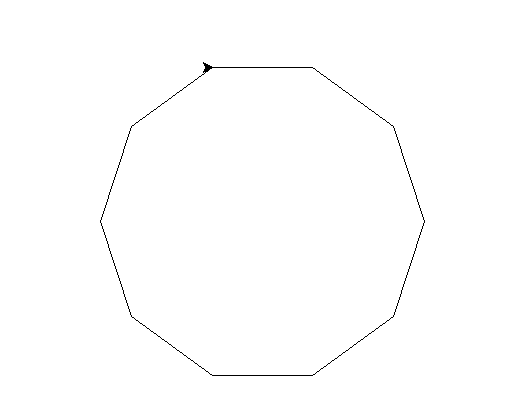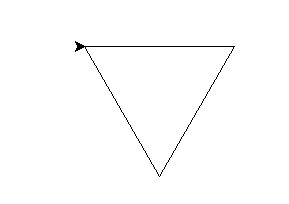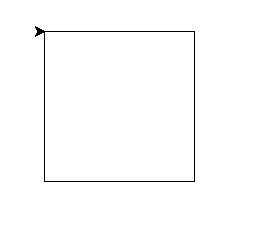# Draw any polygon in Turtle – Python

Prerequisite: Python Turtle Basics

Turtle is an inbuilt module of python. It enables us to draw any drawing by a turtle and methods defined in the turtle module and by using some logical loops. turtle drawings are basically drawn using four methods defined in the turtle module.

forward(x): moves the turtle(pen) in the forward direction by x unit.

backward(x): moves the turtle(pen) in the backward direction by x unit.

right(n): rotate the turtle(pen) by n degree in clockwise direction.

left(n): rotate the turtle(pen) by n degree in anticlockwise direction.

In this article, we will learn how to draw different shaped Polygons using Turtle module. Given the number of sides (n) and length of sides (l), one can easily draw any polygon shape. Let’s try to understand it better with the help of examples.

 `# draw any polygon in turtle ` ` `  `import` `turtle ` ` `  `# creating turtle pen ` `t ``=` `turtle.Turtle() ` ` `  `# taking input for the no of the sides of the polygon ` `n ``=` `int``(``input``(``"Enter the no of the sides of the polygon : "``)) ` ` `  `# taking input for the length of the sides of the polygon ` `l ``=` `int``(``input``(``"Enter the length of the sides of the polygon : "``)) ` ` `  ` `  `for` `_ ``in` `range``(n): ` `    ``turtle.forward(l) ` `    ``turtle.right(``360` `/` `n) `

Input :

```10
100```

Output :Input :

```3
150```

Output :Input :

```4
150```

Output :Whether you're preparing for your first job interview or aiming to upskill in this ever-evolving tech landscape, GeeksforGeeks Courses are your key to success. We provide top-quality content at affordable prices, all geared towards accelerating your growth in a time-bound manner. Join the millions we've already empowered, and we're here to do the same for you. Don't miss out - check it out now!

Previous
Next